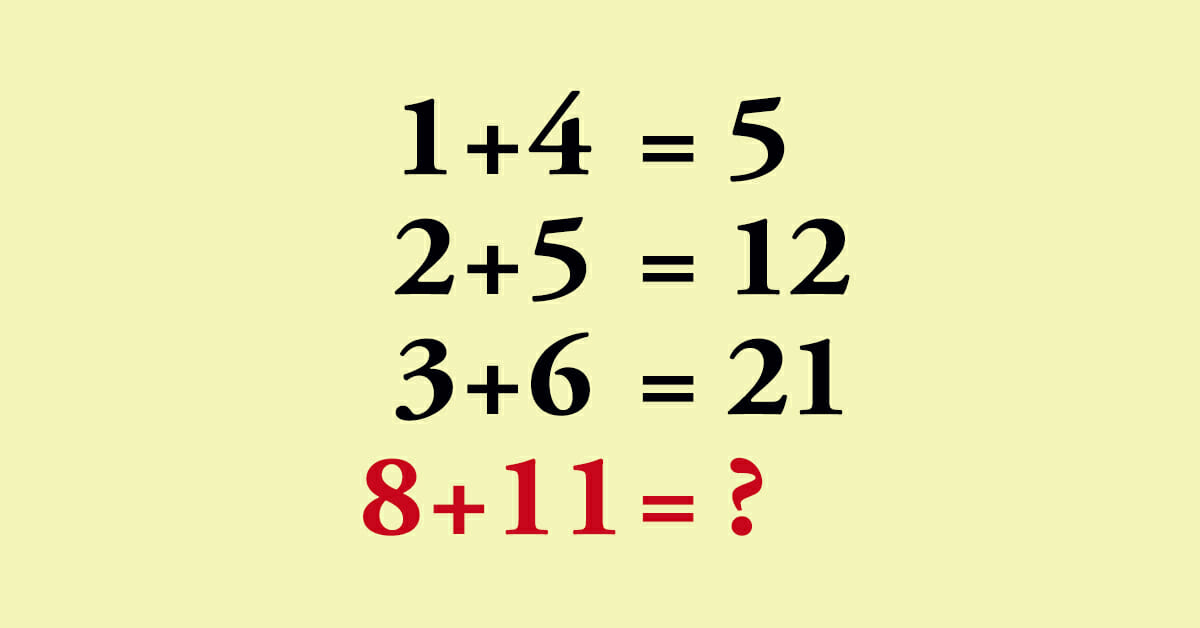# High IQ math puzzle has people scratching their heads – are you smart enough to solve it?

Ask anyone who knows me, and they’ll tell you that math makes my brain hurt.

I know I’m not alone in this, just as I know there are plenty of people in the world who would be perfectly happy if they never had to look at a math equation again in their life.

That said, I find it simply impossible to stay away from online puzzles and brain teasers. The rise of social media has made it far easier to share these oft-frustrating conundrums, resulting in a fair few of them achieving viral fame.

Well, we think we’ve stumbled upon one that could challenge even the most well-versed of budding brain-boxes. There are two solutions to the puzzle, but be warned … neither are that easy to come by.The thing I like most about this particular puzzle is that it provides a reminder just because someone thinks differently to you, they are not necessarily wrong.

So, have a crack at it below, and be sure to let us know what solution you used and what answer it gave you!

Solution number 1

If you used this solution to work out the problem then you’re most likely a straight-forward thinker. You like to interpret things as clearly as possible. If you can steer away from complexity you will.

So … 1 + 4 = 5 (yes, the universe still makes sense, for now)

But then in this equation 2 + 5 = 7, which doesn’t abide by any laws of arithmetics. Of course, those more observant amongst you will have noted that the answers from these first two questions added together will give you the answer you should normally get … 12.

Once you’ve stumbled upon that realization, the rest should follow with a degree of ease. The next equation states that 3 + 6 = 21, which again we know to be completely wrong. Add the supposedly correct answer to the result of the previous expression, though, and you’ll have the number you’re looking for (9 + 12).

And now for the main course … 8 + 11, which makes 19. Add 21 to 19, and you’ll get the answer to the missing equation: 40.

Solution number 2

So, on to the second way of doing it, and one that will have given you a different answer. It’s fair to say this route can be considered a tad more complex, and so could indicate a different way of thinking if this is the one you went for.

In this one, you get the desired answer by multiplying the two given numbers and then adding the first number onto the answer. So in the first instance, 1 x 4 = 4, then 4 + 1 = 5.

2 + 5 = 12, because 2 x 5 is 10, plus the 2 = 12.

3 + 6 = 21, because 3 x 6 = 18, plus the 3 = 21.

8 + 11 = 96, because 8 x 11 is 88, plus the 8 = 96. This makes 96 also a potential answer.

Either one of these answers (40 and 96) are correct, so well done to you if you arrived at those numbers.